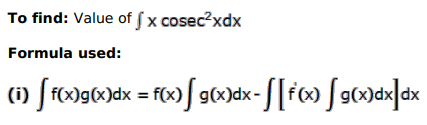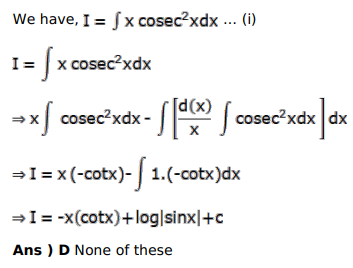# Mark against the correct answer in each of the following:

Question:

Mark $(\sqrt{)}$ against the correct answer in each of the following:

$\int x \operatorname{cosec}^{2} x d x=?$

A. $x \cot x-\log |\sin x|+C$

B. $-\cot x+\log |\sin x|+C$

C. $x \tan x-\log |\sec x|+C$

D. none of these

Solution: